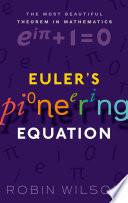# Euler's Pioneering Equation: The Most Beautiful Theorem In Mathematics

Free shipping (Guaranteed delivery 3-5 business days for In-Stock products)
Out of stock

## ISBN details

• ISBN 10: 0198794924
• ISBN 13: 9780198794929

## Overview

In 1988 The Mathematical Intelligencer, a quarterly mathematics journal, carried out a poll to find the most beautiful theorem in mathematics. Twenty-four theorems were listed and readers were invited to award each a 'score for beauty'. While there were many worthy competitors, the winner was 'Euler's equation'. In 2004 Physics World carried out a similar poll of 'greatest equations', and found that among physicists Euler's mathematical result came second only to Maxwell's equations. The Stanford mathematician Keith Devlin reflected the feelings of many in describing it as "like a Shakespearian sonnet that captures the very essence of love, or a painting which brings out the beauty of the human form that is far more than just skin deep, Euler's equation reaches down into the very depths of existence." What is it that makes Euler's identity, e]iPi + 1 = 0, so special? In Euler's Pioneering Equation Robin Wilson shows how this simple, elegant, and profound formula links together perhaps the five most important numbers in mathematics, each associated with a story in themselves: the number 1, the basis of our counting system; the concept of zero, which was a major development in mathematics, and opened up the idea of negative numbers; Pi an irrational number, the basis for the measurement of circles; the exponential e, associated with exponential growth and logarithms; and the imaginary number i, the square root of -1, the basis of complex numbers. Following a chapter on each of the elements, Robin Wilson discusses how the startling relationship between them was established, including the several near misses to the discovery of the formula.

## Other Details

• Publisher: Oxford University Press
• Pages: 162
• Date Published: 2018
• Authors: Wilson, Robin